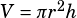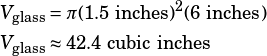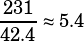Test 4 Section 4 Number 18

This is a volume question. The first thing we need to do is figure out the volume of liquid each of these glasses will hold. You need not have any formulas memorized—they’re in the beginning of the section. The volume of a right circular cylinder can be found using this formula:.

In this case, we’re told that the height is 6 inches and the internal diameter is 3 inches. If the diameter is 3 inches, then the radius is 1.5 inches. So the volume of the glasses:He has 231 cubic inches—how many glasses can he fill all the way up?If he can fill 5.4 glasses, then he can only fill 5 all the way up.